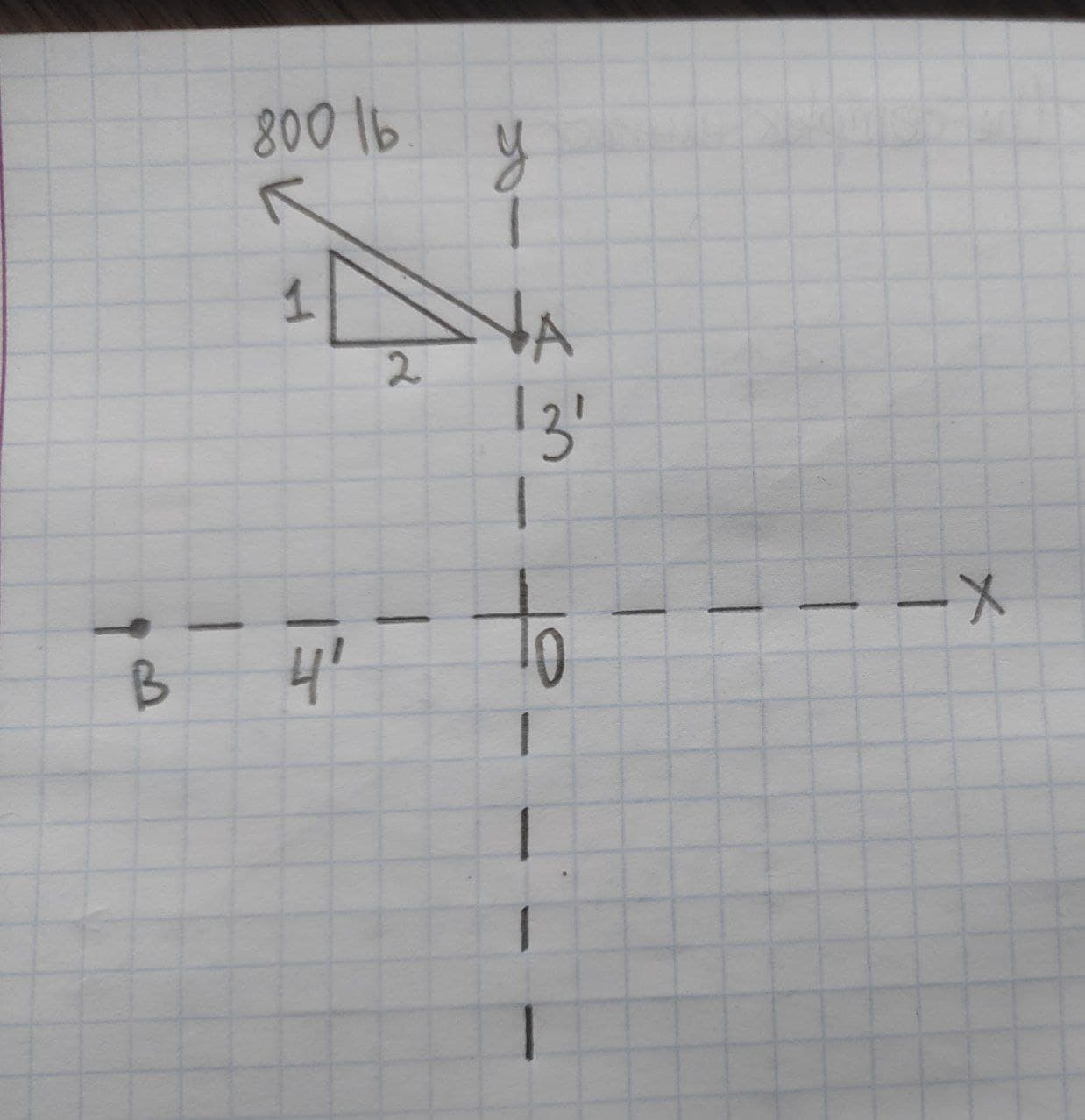# What is 12(cos 60 + i sin 60)Question
Complex numbersWhat is $$\displaystyle{12}{\left({\cos{{60}}}+{i}{\sin{{60}}}\right)}$$2020-10-22
$$\displaystyle{12}{\left({\cos{{60}}}+{i}{\sin{{60}}}\right)}={12}{\left(\frac{{1}}{{2}}+{i}\frac{\sqrt{{3}}}{{2}}\right)}={6}+{6}{i}\sqrt{{3}}={6}{\left({1}+{i}\sqrt{{3}}\right)}$$.

### Relevant QuestionsWrite the standard form of the complex number.
$$\displaystyle{12}{\left({\cos{{\left({60}^{\circ}\right)}}}+{i}{\sin{{\left({60}^{\circ}\right)}}}\right)}$$Is the expression true:
$$\displaystyle\frac{{{\cos{{\left(-\theta\right)}}}}}{{{1}+{\sin{{\left(-\theta\right)}}}}}={\sec{\theta}}+{\tan{\theta}}$$A bird flies in the xy-plane with a position vector given by $$\displaystyle\vec{{{r}}}={\left(\alpha{t}-\beta{t}^{{3}}\right)}\hat{{{i}}}+\gamma{t}^{{2}}\hat{{{j}}}$$, with $$\displaystyle\alpha={2.4}\ \frac{{m}}{{s}},\beta={1.6}\ \frac{{m}}{{s}^{{3}}}$$ and $$\displaystyle\gamma={4.0}\ \frac{{m}}{{s}^{{2}}}$$. The positive y-direction is vertically upward. At the bird is at the origin.
Calculate the velocity vector of the bird as a function of time.
Calculate the acceleration vector of the bird as a function oftime.
What is the bird's altitude(y-coordinate) as it flies over x=0 for the first time after ?Which operation could we perform in order to find the number of milliseconds in a year?
$$\displaystyle{60}\cdot{60}\cdot{24}\cdot{7}\cdot{365}$$
$$\displaystyle{1000}\cdot{60}\cdot{60}\cdot{24}\cdot{365}$$
$$\displaystyle{24}\cdot{60}\cdot{100}\cdot{7}\cdot{52}$$
$$\displaystyle{1000}\cdot{60}\cdot{24}\cdot{7}\cdot{52}?$$Write each complex number in standard form. $$\displaystyle{2}{\left({{\cos{{30}}}^{\circ}+}{i}{\sin{{30}}}^{\circ}\right)}$$Write the complex number in trigonometric form r(cos theta + i sin theta),with theta in the interval $$\displaystyle{\left[{0}^{\circ},{360}^{\circ}\right]}$$
$$\displaystyle{5}\sqrt{{3}}+{5}{i}$$Which statement is correct?
$$\displaystyle\frac{{{3.56}\cdot{10}^{{2}}}}{{{1.09}\cdot{10}^{{4}}}}\le{\left({4.08}\cdot{10}^{{2}}\right)}{\left({1.95}\cdot{10}^{{-{{6}}}}\right)}$$
$$\displaystyle\frac{{{3.56}\cdot{10}^{{2}}}}{{{1.09}\cdot{10}^{{4}}}}{<}{\left({4.08}\cdot{10}^{{2}}\right)}{\left({1.95}\cdot{10}^{{-{{6}}}}\right)}$$
$$\displaystyle\frac{{{3.56}\cdot{10}^{{2}}}}{{{1.09}\cdot{10}^{{4}}}}{>}{\left({4.08}\cdot{10}^{{2}}\right)}{\left({1.95}\cdot{10}^{{-{{6}}}}\right)}$$
$$\displaystyle\frac{{{3.56}\cdot{10}^{{2}}}}{{{1.09}\cdot{10}^{{4}}}}={\left({4.08}\cdot{10}^{{2}}\right)}{\left({1.95}\cdot{10}^{{-{{6}}}}\right)}$$$$\displaystyle{9}^{{x}}-{3}^{{{x}+{1}}}+{1}={0}$$